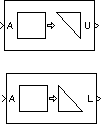Documentation

Extract Triangular Matrix

Extract lower or upper triangle from input matrices

Library

Math Functions / Matrices and Linear Algebra / Matrix Operations

dspmtrx3Description

The Extract Triangular Matrix block creates a triangular matrix output from the upper or lower triangular elements of an M-by-N input matrix. The block treats length-M unoriented vector inputs as an M-by-1 matrix.

The Extract parameter selects between the two components of the input:

• Upper — Copies the elements on and above the main diagonal of the input matrix to an output matrix of the same size. The first row of the output matrix is therefore identical to the first row of the input matrix. The elements below the main diagonal of the output matrix are zero.

• Lower — Copies the elements on and below the main diagonal of the input matrix to an output matrix of the same size. The first column of the output matrix is therefore identical to the first column of the input matrix. The elements above the main diagonal of the output matrix are zero.

Examples

The ex_extracttriang_ref model below shows the extraction of upper and lower triangles from a 5-by-3 input matrix.Parameters

Extract

The component of the matrix to copy to the output: upper triangle or lower triangle.

Simulate using

Code generation (default) — Simulate model using generated C code. The first time you run a simulation, Simulink® generates C code for the block. The C code is reused for subsequent simulations, as long as the model does not change. This option requires additional startup time but provides faster simulation speed than Interpreted execution.

Interpreted execution — Simulate model using the MATLAB® interpreter. This option shortens startup time but has slower simulation speed than Code generation.

Supported Data Types

PortSupported Data Types

A

• Double-precision floating point

• Single-precision floating point

• Fixed point (signed and unsigned)

• Boolean

• 8-, 16-, and 32-bit signed integers

• 8-, 16-, and 32-bit unsigned integers

U

• Double-precision floating point

• Single-precision floating point

• Fixed point (signed and unsigned)

• Boolean

• 8-, 16-, and 32-bit signed integers

• 8-, 16-, and 32-bit unsigned integers

L

• Double-precision floating point

• Single-precision floating point

• Fixed point (signed and unsigned)

• Boolean

• 8-, 16-, and 32-bit signed integers

• 8-, 16-, and 32-bit unsigned integers

 Autocorrelation LPC DSP System Toolbox Cholesky Factorization DSP System Toolbox Extract Diagonal DSP System Toolbox Forward Substitution DSP System Toolbox LDL Factorization DSP System Toolbox LU Factorization DSP System Toolbox tril MATLAB triu MATLAB

Watch now